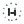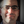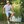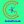0%
0%

# Digital Arduino Voltmeter

A Voltmeter or a Voltage Meter is a measuring instrument that is used for measuring voltage.

Similar projects worth following
0 followers
To overcome the drawbacks of analog voltmeters, we have designed a digital voltmeter based on Arduino. Rather than only scaling and pointing to show a measured voltage like analog voltmeter, digital voltmeters directly display the measured voltage on the digital display.

Circuit Design

Pin 1 and Pin 2 (Vss and Vdd) of the LCD power supply are the pins for display. They are attached to ground and +5V supply respectively. Pin 3 (Vee) of the LCD is connected to the wiper terminal of the 10KΩ POT and the other terminals of the POT are connected to +5V supply and ground respectively.

The next 3 pins of the LCD are control pins. Pin 4 and Pin 6 of the LCD are attached to digital input/output pins 2 and 3 of Arduino respectively. Pin 5 (RW) of the LCD is attached to the ground.

Pin 15 (LED+) of the LCD is connected to +5V supply via a current limiting resistor of 220Ω. Pin 16 (LED-) of the LCD is attached to the ground.

The output of the voltage divider circuit consisting of 100KΩ resistor and 10KΩ resistor is attached to the analog input pin A0 of the Arduino UNO with another end of the 100KΩ resistor attached to the voltage to be calculated and the other end of the 10KΩ resistor attached to the ground.

Working

In a digital voltmeter, the voltages to be estimated, which are in analog form, are switched to digital form with the help of Analog to Digital Converters (ADC). Hence, the ADC specialty of the Arduino UNO is used in this project.

The span of voltages for Arduino Uno's analog input is 0V to 5V. Hence, in order to improve this range, a voltage divider circuit need to be used.

With the help of the voltage divider circuit, the input voltage being calculated is taken down to the range of Arduino UNOs analog input.

With the help of IoT Course it is easy to build an Customized IoT Solutions.

• 1 × Arduino Uno
• 1 × 16x2 LCD
• 1 × Single Turn Potentiometer- 10k ohms Non-IC RF, IF, RFID, ZigBee Components / Inductors and Coils
• 1 × Resistor 100k ohm
• 1 × Resistor 10k ohm

• ### Run a Code

```/*
DC Voltmeter */
#include LiquidCrystal lcd(7, 8, 9, 10, 11, 12);
float vout = 0.0;
float vin = 0.0;
float R1 = 100000.0; // resistance of R1 (100K) -see text!
float R2 = 10000.0; // resistance of R2 (10K) - see text!
int value = 0;
void setup(){   pinMode(analogInput, INPUT);   lcd.begin(16, 2);   lcd.print("DC VOLTMETER");
}
void loop(){   // read the value at analog input   value = analogRead(analogInput);   vout = (value * 5.0) / 1024.0; // see text   vin = vout / (R2/(R1+R2));    if (vin<0.09) {   vin=0.0;//statement to quash undesired reading !
} lcd.setCursor(0, 1);
lcd.print("INPUT V= ");
lcd.print(vin);
delay(500);
}```

Share

## Discussions

Ken Yap wrote 12/03/2019 at 10:18 point

>A Voltmeter or a Voltage Meter is a measuring instrument that is used for measuring voltage.

I'm sure you could also submit this description for a pleonasm award. 😉

Are you sure? yes | no

## Similar Projects

Project Owner Contributor

### Arduino Based Digital ThermometerhIOTron

Project Owner Contributor

### Arduino LCD Temperature ControllerTimothy Coyle

Project Owner Contributor

### Digital Battery Operated PowersupplyThomas Van den Dries

Project Owner Contributor

### A bipolar analog I/O for Arduino DueGiovanni Carrera

# Does this project spark your interest?

Become a member to follow this project and never miss any updates